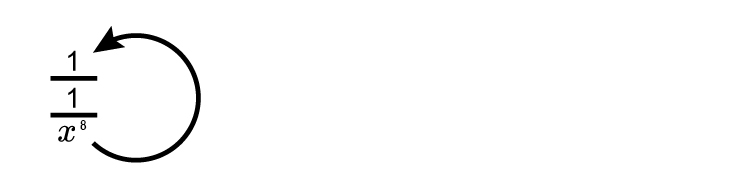# Indices and double negatives

Simplify  (x^-4)^-2

To tackle this problem try simple numbers you know first.

We know

10^-2=1/10^2=1/(10\times10)=1/100=0.01

Therefore  x^(-4)=1/(x^4)

And therefore  (x^-4)^-2=(1/x^4)^-2

Which equals  1/((1/(x^4))^2

And  1/{1/x^4\times1/x^4

And as  1/2\times1/2=1/4

Then 1/{1/x^4\times1/x^4\}=1/{1/x^8}x^8/1

We get  x^8

(x^-4)^-2=\x^8